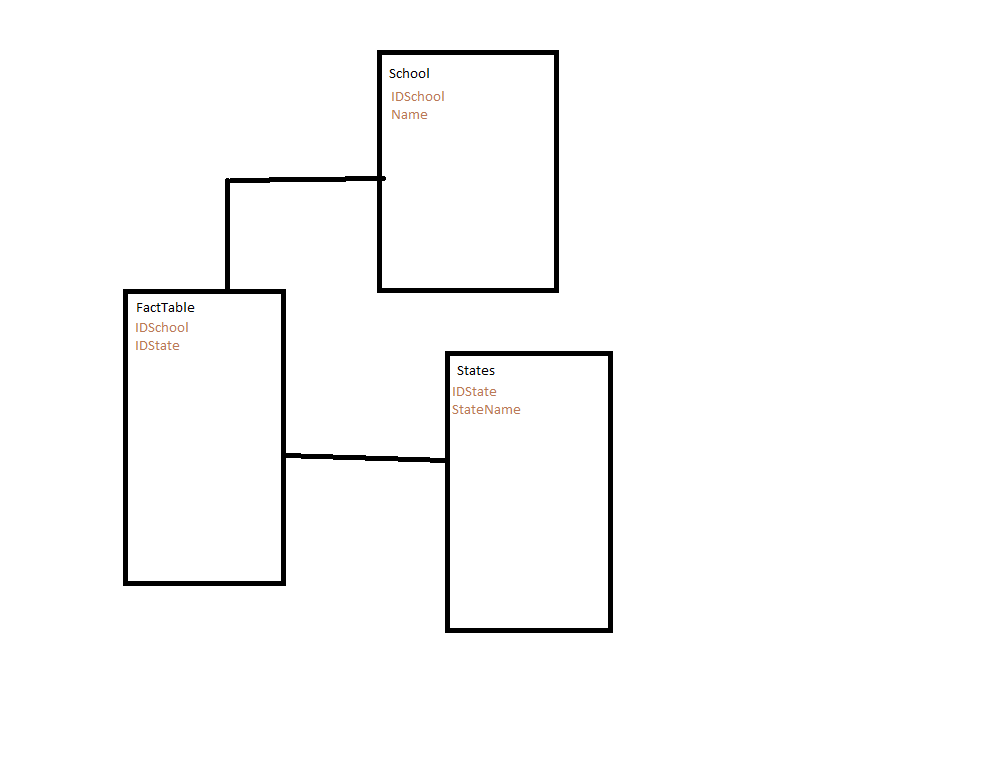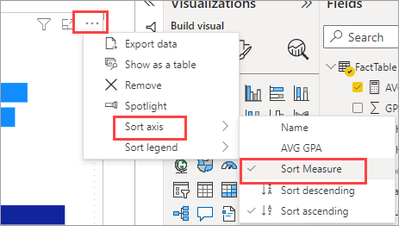cancel
Showing results for
Did you mean:Frequent Visitor

## Sort bar chart by category

Hi everyone !

I have a bar chart like this :I'd like to have all the pink together, then all the blue, etc on the same bar chart, while keeping the current order within a given color group.

If you need more context, these are average GPAs of different schools, color coded by states. And here is my model :Thank you !

1 ACCEPTED SOLUTIONSuper User

You can sort a chart using a measure that is not on the chart by adding the measure to the tooltip.Then setting the sort on the chart to use the measure:Now the sort measure.  First it calcualtes an alphabetical ranking of the states and multiplies that by 100 then adds the ranking of the school based on [Average GPA].  The * 100 + school ranking returns something like:
All Arizona schools are ranked 1__ then the shool ranking is added on so we get 101, 102, 103 for Arizona schools, 201, 202, 203 for California schools, etc.

``````Sort Measure =
DIVIDE ( [AVG GPA], [AVG GPA] ) *
(
RANKX(ALL(FactTable),CALCULATE(MAX(FactTable[State])),,ASC,Dense) * 100 +
RANKX(ALL(School),[AVG GPA],,DESC,Dense)
)``````

The
DIVIDE ( [AVG GPA], [AVG GPA] ) *

Keeps the ranking from calculating where there is no GPA.
I have attached my sample file for you to look at.

2 REPLIES 2Super User

You can sort a chart using a measure that is not on the chart by adding the measure to the tooltip.Then setting the sort on the chart to use the measure:Now the sort measure.  First it calcualtes an alphabetical ranking of the states and multiplies that by 100 then adds the ranking of the school based on [Average GPA].  The * 100 + school ranking returns something like:
All Arizona schools are ranked 1__ then the shool ranking is added on so we get 101, 102, 103 for Arizona schools, 201, 202, 203 for California schools, etc.

``````Sort Measure =
DIVIDE ( [AVG GPA], [AVG GPA] ) *
(
RANKX(ALL(FactTable),CALCULATE(MAX(FactTable[State])),,ASC,Dense) * 100 +
RANKX(ALL(School),[AVG GPA],,DESC,Dense)
)``````

The
DIVIDE ( [AVG GPA], [AVG GPA] ) *

Keeps the ranking from calculating where there is no GPA.
I have attached my sample file for you to look at.Frequent Visitor

That's absolutely perfect, thank you so much !

My Average GPA was actually not a measure but a field in the FactTable (forgot to show it in my simplified model) but using

``MAX(FactTable[Average GPA])``

instead of just [Average GPA] solved that issue 🙂